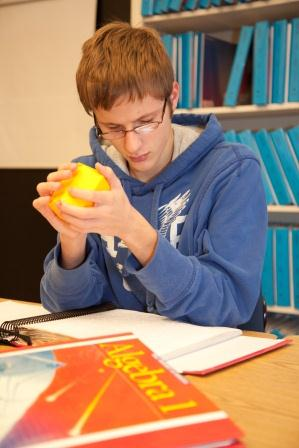### What is Mathematical Literacy?Mathematical Literacy includes numeracy (the ability to recognize numerals), a basic number sense, and a grasp of simple mathematical concepts.  Students who are blind or visually impaired often do not have the same exposure to numbers or mathematical concepts as their sighted peers and, as a result, they may be behind their age-level peers in school.

#### Basic Concepts

An understanding of math begins with the development of basic concepts, such as the attributes of size (big/little) and weight (heavy/light); same/different, comparison (bigger/smaller), more/less.  Color and shape recognition (3D and 2D) are other early concepts that are critical to the development of mathematical skills.  Recognizing and imitating patterns is another important early concept, whether the patterns be tactile, visual or both (e.g. rough, rough, smooth or circle, square, triangle, circle, square, triangle).

#### Counting and Number Sense

Counting begins with rote counting, which can later be expanded to counting by 2s, 5s, 10s, 100s.  An understanding of one-to-one correspondence is necessary to the ability to count items.  Counting is a skill which requires the ability to count rotely, as well as the ability to make smaller sets from larger sets (e.g. Given 5 spoons, the child is asked to give 3.  Initially children will give all 5, and it’s only when they have a clear grasp of number sense that they can make smaller sets out of a larger array.

#### Abacus

One of the specialized tools for computing is an adapted abacus, known as the Cranmer abacus.  This device of moveable beads on metal rods, like the ancient abacus that has been used for centuries, can be used to perform mathematical functions of addition, subtraction, multiplication, division, square root and cube root. It is often introduced in the early elementary grades, although it can be used at any age. Skilled abacus users can complete even complex mathematical problems quickly, and use the abacus in the same way that sighted students will use pencil and paper.  This tool requires mathematical knowledge, unlike a calculator which performs functions simply by pushing the correct keys.

#### Functional Math Skills

Functional Math Skills include a grasp of concepts that are used in daily life, such as money, measurement, budgeting, and estimation.  Teaching these skills in the context of cooking, shopping, and developing independent living skills can reinforce these skills.

Digitally Accessible Math Worksheets

Many math worksheets are not accessible to students who use screen readers.  Check out our digitally-accessible worksheets for students in grades 5-8.

### Nemeth Code for Braille Readers

Just as sighted students must see numerals and basic mathematical symbols (+, -, =, > <) before they begin to use them, so too must students who are braille readers have exposure to Nemeth Code, which is the braille code used to transcribe mathematics, science, and other technical information.  For more information about Nemeth Code, see the following: